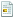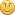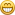October 06, 2022, 10:28:16 AM

## News:

Own IWBasic 2.x ? -----> Get your free upgrade to 3.x now.........

## Problem with DELETE

Started by billhsln, April 05, 2010, 12:10:43 PM

0 Members and 1 Guest are viewing this topic.

#### billhsln

#####April 05, 2010, 12:10:43 PM
I am able to CREATE the table, INSERT records into the table, and UPDATE records in the table.  However, when I try to DELETE records I get 42000 error.

Here is the DELETE code I am using:

`temp = "DELETE FROM " + UserID + " WHERE "temp += "n_ex = 0 AND n_g = AND n_p = 0 AND "temp += "r_ex = 0 AND r_g = AND r_p = 0"hStmt = dbExecSQL(pDB,temp)error = dbGetErrorCode(hStmt)IF LEN(error) MESSAGEBOX d1,"problem with DELETE '" + UserID + "' " + error,"Error" co = 1 OPENCONSOLE PRINT LEN(temp) PRINT temp,"-"ENDIFdbFreeSQL(hStmt)`

Here is my INSERT + UPDATE code:

`hStmt = dbExecSQL(pDB,"INSERT INTO " + UserID + " (recno,n_ex,n_g,n_p,r_ex,r_g,r_p) VALUES(" + STR\$(RecID) + "," + STR\$(e1) + "," + STR\$(e2) + "," + STR\$(e3) + "," + STR\$(e4) + "," + STR\$(e5)  + "," + STR\$(e6)+ ")")error = dbGetErrorCode(hStmt)IF LEN(error) dbFreeSQL(hStmt) hStmt = 0 IF error <> "23000" MESSAGEBOX d1,"Problem with INSERT '" + UserID + "' " + error,"Error" ELSE SELECT fld CASE 1 hStmt = dbExecSQL(pDB,"UPDATE " + UserID + " SET n_ex =" + STR\$(e1) + " WHERE recno = " + STR\$(RecID)) CASE 2 hStmt = dbExecSQL(pDB,"UPDATE " + UserID + " SET n_g =" + STR\$(e2) + " WHERE recno = " + STR\$(RecID)) CASE 3 hStmt = dbExecSQL(pDB,"UPDATE " + UserID + " SET n_p =" + STR\$(e3) + " WHERE recno = " + STR\$(RecID)) CASE 4 hStmt = dbExecSQL(pDB,"UPDATE " + UserID + " SET r_ex =" + STR\$(e4) + " WHERE recno = " + STR\$(RecID)) CASE 5 hStmt = dbExecSQL(pDB,"UPDATE " + UserID + " SET r_g =" + STR\$(e5) + " WHERE recno = " + STR\$(RecID)) CASE 6 hStmt = dbExecSQL(pDB,"UPDATE " + UserID + " SET r_p =" + STR\$(e6) + " WHERE recno = " + STR\$(RecID)) ENDSELECT error = dbGetErrorCode(hStmt) IF LEN(error) dbFreeSQL(hStmt) MESSAGEBOX d1,"Problem with UPDATE '" + UserID + "' " + error,"Error" hStmt = 0 RETURN ENDIF ENDIFENDIFdbFreeSQL(hStmt)`

I would assume that I have full access to the file, but keep getting 42000 error when doing the DELETE.

Any help will be appreciated.

Thanks,
Bill
When all else fails, get a bigger hammer.

#### LarryMc

#####April 05, 2010, 12:45:04 PM #1
Bill
Just glancing at it, these look wrong:
`temp += "n_ex = 0 AND n_g = AND n_p = 0 AND "temp += "r_ex = 0 AND r_g = AND r_p = 0"`

maybe should be:
`temp += "n_ex = 0 AND n_g = 0  AND n_p = 0 AND "temp += "r_ex = 0 AND r_g = 0 AND r_p = 0"`

LarryMc
LarryMc
Larry McCaughnAuthor of IWB+, Custom Button Designer library, Custom Chart Designer library, Snippet Manager, IWGrid control library, LM_Image control library

#### billhsln

#####April 05, 2010, 12:56:13 PM #2
Thank you, Larry.I have been looking at this problem for about 2 hours and kept missing the obvious.

Some times it just takes another pair of eyes to see what you are missing.

Thanks again,
Bill
When all else fails, get a bigger hammer.

#### REDEBOLT

#####April 06, 2010, 10:09:16 AM #3
Just by looking at your code, I see the following:

temp += "n_ex = 0 AND n_g = AND n_p = 0 AND "
temp += "r_ex = 0 AND r_g = AND r_p = 0"

It appears that n_g and r_g are not assigned a value.
Regards,
Bob